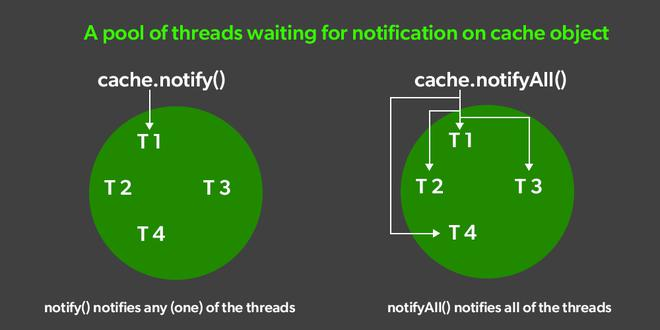Open in App
Not now

# Difference Between notify() and notifyAll() in Java

• Difficulty Level : Expert
• Last Updated : 09 Nov, 2022

The notify() and notifyAll() methods with wait() methods are used for communication between the threads. A thread that goes into waiting for state by calling the wait() method will be in waiting for the state until any other thread calls either notify() or notifyAll() method on the same object.

notify(): The notify() method is defined in the Object class, which is Java’s top-level class. It’s used to wake up only one thread that’s waiting for an object, and that thread then begins execution. The thread class notify() method is used to wake up a single thread.

notifyAll(): The notifyAll() wakes up all threads that are waiting on this object’s monitor. A thread waits on an object’s monitor by calling one of the wait methods. The awakened threads will not be able to proceed until the current thread relinquishes the lock on this object.Now the question is both notify() and notifyAll() the method is used to give notification to the waiting thread, then what is the difference between them or where we should use notify() method and where we should go for the notifyAll() method?

Let’s understand how the notify() method behaves:

## Java

 `// Java program to illustrate the``// behaviour of notify() method``class` `Geek1 ``extends` `Thread {``    ``public` `void` `run()``    ``{``        ``synchronized` `(``this``)``        ``{``            ``System.out.println(``                ``Thread.currentThread().getName()``                ``+ ``"...starts"``);``            ``try` `{``                ``this``.wait();``            ``}``            ``catch` `(InterruptedException e) {``                ``e.printStackTrace();``            ``}``            ``System.out.println(``                ``Thread.currentThread().getName()``                ``+ ``"...notified"``);``        ``}``    ``}``}``class` `Geek2 ``extends` `Thread {``    ``Geek1 geeks1;``  ` `    ``Geek2(Geek1 geeks1){``      ``this``.geeks1 = geeks1;``    ``}``  ` `    ``public` `void` `run()``    ``{``        ``synchronized` `(``this``.geeks1)``        ``{``            ``System.out.println(``                ``Thread.currentThread().getName()``                ``+ ``"...starts"``);` `            ``try` `{``                ``this``.geeks1.wait();``            ``}``            ``catch` `(InterruptedException e) {``                ``e.printStackTrace();``            ``}``            ``System.out.println(``                ``Thread.currentThread().getName()``                ``+ ``"...notified"``);``        ``}``    ``}``}``class` `Geek3 ``extends` `Thread {``    ``Geek1 geeks1;``    ``Geek3(Geek1 geeks1) { ``this``.geeks1 = geeks1; }``    ``public` `void` `run()``    ``{``        ``synchronized` `(``this``.geeks1)``        ``{``            ``System.out.println(``                ``Thread.currentThread().getName()``                ``+ ``"...starts"``);``            ``this``.geeks1.notify();``            ``System.out.println(``                ``Thread.currentThread().getName()``                ``+ ``"...notified"``);``        ``}``    ``}``}``class` `MainClass {``    ``public` `static` `void` `main(String[] args)``        ``throws` `InterruptedException``    ``{` `        ``Geek1 geeks1 = ``new` `Geek1();``        ``Geek2 geeks2 = ``new` `Geek2(geeks1);``        ``Geek3 geeks3 = ``new` `Geek3(geeks1);``        ``Thread t1 = ``new` `Thread(geeks1, ``"Thread-1"``);``        ``Thread t2 = ``new` `Thread(geeks2, ``"Thread-2"``);``        ``Thread t3 = ``new` `Thread(geeks3, ``"Thread-3"``);``        ``t1.start();``        ``t2.start();``        ``Thread.sleep(``100``);``        ``t3.start();``    ``}``}`

Output:

```Thread-1...start

Lets understand how notifyAll() method behaves:

## Java

 `// Java program to illustrate the``// behavior of notifyAll() method` `class` `Geek1 ``extends` `Thread {``    ``public` `void` `run()``    ``{``        ``synchronized` `(``this``)``        ``{``            ``System.out.println(``                ``Thread.currentThread().getName()``                ``+ ``"...starts"``);``            ``try` `{``                ``this``.wait();``            ``}``            ``catch` `(InterruptedException e) {``                ``e.printStackTrace();``            ``}``            ``System.out.println(``                ``Thread.currentThread().getName()``                ``+ ``"...notified"``);``        ``}``    ``}``}``class` `Geek2 ``extends` `Thread {``    ``Geek1 geeks1;``  ` `    ``Geek2(Geek1 geeks1){``      ``this``.geeks1 = geeks1;``    ``}``  ` `    ``public` `void` `run()``    ``{``        ``synchronized` `(``this``.geeks1)``        ``{``            ``System.out.println(``                ``Thread.currentThread().getName()``                ``+ ``"...starts"``);` `            ``try` `{``                ``this``.geeks1.wait();``            ``}``            ``catch` `(InterruptedException e) {``                ``e.printStackTrace();``            ``}``            ``System.out.println(``                ``Thread.currentThread().getName()``                ``+ ``"...notified"``);``        ``}``    ``}``}``class` `Geek3 ``extends` `Thread {``    ``Geek1 geeks1;``    ``Geek3(Geek1 geeks1) { ``this``.geeks1 = geeks1; }``    ``public` `void` `run()``    ``{``        ``synchronized` `(``this``.geeks1)``        ``{``            ``System.out.println(``                ``Thread.currentThread().getName()``                ``+ ``"...starts"``);` `            ``this``.geeks1.notifyAll();``            ``System.out.println(``                ``Thread.currentThread().getName()``                ``+ ``"...notified"``);``        ``}``    ``}``}``class` `MainClass {``    ``public` `static` `void` `main(String[] args)``        ``throws` `InterruptedException``    ``{` `        ``Geek1 geeks1 = ``new` `Geek1();``        ``Geek2 geeks2 = ``new` `Geek2(geeks1);``        ``Geek3 geeks3 = ``new` `Geek3(geeks1);``        ``Thread t1 = ``new` `Thread(geeks1, ``"Thread-1"``);``        ``Thread t2 = ``new` `Thread(geeks2, ``"Thread-2"``);``        ``Thread t3 = ``new` `Thread(geeks3, ``"Thread-3"``);``        ``t1.start();``        ``t2.start();``        ``Thread.sleep(``100``);``        ``t3.start();``    ``}``}`

Output

```Thread-1...starts

### When to Use notify() method and notifyAll()?

• In the case of mutually exclusive locking, only one of the waiting threads can do something useful after being notified (in this case acquire the lock). In such a case, you would rather use notify(). Properly implemented, you could use notifyAll() in this situation as well, but you would unnecessarily wake threads that can’t do anything anyway.
• In some cases, all waiting threads can take useful action once the wait finishes. An example would be a set of threads waiting for a certain task to finish; once the task has finished, all waiting threads can continue with their business. In such a case you would use notifyAll() to wake up all waiting threads at the same time.

### Applications of notify() and notifyAll()

• A maintenance operation on a shared resource, where multiple threads are waiting for the operation to complete before accessing the resource; for these, we should go for notifyAll().
• Let’s say we have a producer thread and a consumer thread. Each “packet” produced by the producer should be consumed by a consumer. The consumer puts something in a queue and then calls notify().
• We want to have a notification when a lengthy process has finished. You want a beep and a screen update. The process performs notifyAll() to notify both the beeping thread and the screen-update-thread.